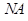Chapter 26, Problem 18CQ

Chapter
Section
Textbook Problem

How does the NA of a microscope compare wi1h the NA of an optical liber?

To determine

The comparison betweenof a microscope with theof an optical fiber.

Explanation

Introduction:

The numerical aperture gives us information about how much light can be captured by an optical system such as optical fiber and microscopes. It is related to the resolving power of a lens.

The numerical aperturecan be expressed as:

Where:

is the refraction index

Still sussing out bartleby?

Check out a sample textbook solution.

See a sample solution

The Solution to Your Study Problems

Bartleby provides explanations to thousands of textbook problems written by our experts, many with advanced degrees!

Get Started